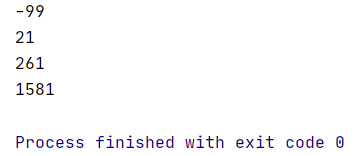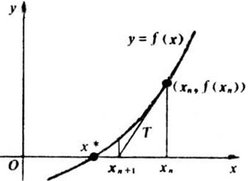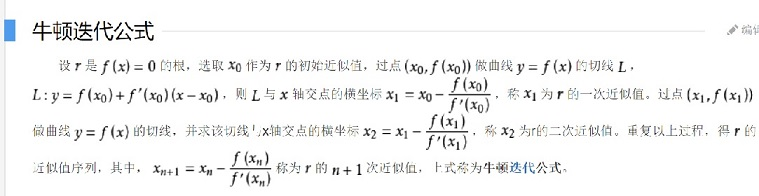• 突然发现我这博客咋啥都开始写了呢。。。 上微积分课胡思乱想系列。...首先，从定义出发，个数aa的kk次方(k∈Zk\in \mathbb{Z})如何定义？ kk个aa连乘。 因为乘法在R\mathbb R上均有定义，所以aa的正整数次方在R\
突然发现我这博客咋啥都开始写了呢。。。
上微积分课胡思乱想系列。。。

显然这个东西在数学上是没有定义的。
包括−1$-1$的13$\frac13$次方这样的东西，数学上的定义也挺模糊的
不过我们可以想想这东西应该怎么定义。。。

首先，从定义出发，一个数a$a$的k$k$次方(k∈Z$k\in \mathbb{Z}$)如何定义？
k$k$个a$a$连乘。
因为乘法在R$\mathbb R$上均有定义，所以a$a$的正整数次方在R$\mathbb R$上均有定义。

接下来，一个数a$a$的负k$k$次方(k∈Z$k\in \mathbb{Z}$)该如何定义？
根据k>0$k>0$时候的定义有ak1∗ak2=ak1+k2$a^{k_1}*a^{k_2}=a^{k_1+k_2}$，因此定义应该将这个性质延续下去，即a−k∗ak+1=a−k+k+1=a$a^{-k}*a^{k+1}=a^{-k+k+1}=a$，a−k=aak+1=1ak$a^{-k}=\frac a{a^{k+1}}=\frac1{a^k}$
据此也有a0=ak∗a−k=1$a^0=a^k*a^{-k}=1$
1/0$1/0$无定义，因此以上二者的定义域都是a∈{x|x∈R,x≠0}$a\in \{x|x\in\mathbb R,x\neq0\}$

整数域已经做完了，下面我们该进入有理数域了。

一个数a$a$的pq$\frac pq$次方等于多少？
和上面类似，根据整数时候的定义有(ak1)k2=ak1k2$(a^{k_1})^{k_2}=a^{k_1k_2}$，因此新的定义应该将这个性质延续下去，即(ap/q)q=ap$(a^{p/q})^q=a^p$
即ap/q$a^{p/q}$是ap$a^p$的q$q$次方根

什么是ap$a^p$的q$q$次方根？
定义ap$a^p$的q$q$次方根为方程xq=ap$x^q=a^p$的解
当q$q$为奇数的时候这个方程在R$\mathbb R$上有唯一解，但q$q$为偶数的时候，当ap≥0$a^p\geq0$时在R$\mathbb R$上有两个解，当ap$a^p$为负数的时候在R$\mathbb R$上无解！
为了保护一些函数的优雅性质，当ap≥0$a^p\geq0$时我们定义了算数次方根为二者中非负的那个，至于ap<0$a^p<0$，我们不讨论了……
可是负数很可怜不是么？
比如(−8)13$(-8)^{\frac13}$按照定义在实数上是有定义的啊……
而且照着这定义来会出事……
比如根据定义，(−8)13$(-8)^{\frac13}$是x3=−8$x^3=-8$在R$\mathbb R$上的唯一解−2$-2$，可是(−8)13=(−8)26$(-8)^{\frac13}=(-8)^{\frac26}$是x6=64$x^6=64$的非负实数解，即2$2$……

这个问题我们放在后面讨论吧。

终于到了无理数了。
显然根据我们已有的运算，我们没什么办法把一个无理数次方的运算搞到有理数域去。

我们的第一想法是利用泰勒展开
设函数
f(x)=(x+1)α=10!+αx1!+α(α−1)x22!+α(α−1)(α−2)x33!+...$f(x)=(x+1)^\alpha=\frac1{0!}+\frac{\alpha x}{1!}+\frac{\alpha(\alpha-1)x^2}{2!}+\frac{\alpha(\alpha-1)(\alpha-2)x^3}{3!}+...$
易证当α$\alpha$为正无理数时这东西收敛当且仅当x∈[−1,1]$x\in[-1,1]$

不过用这个方法我们可以知道正数的无理数次方的定义
以2√$\sqrt2$为例，假设我们想要知道a2√(a>0)$a^\sqrt2(a>0)$的值
若a∈(0,1]$a\in(0,1]$，直接将x=a−1$x=a-1$代入泰勒展开式，其极限即为a2√$a^\sqrt2$
若a∈(1,+∞)$a\in(1,+\infty)$，将x=1a−1$x=\frac1a-1$代入泰勒展开式，其极限的倒数即为a2√$a^\sqrt2$
由此我们得到了实数域上正数的无理数次方的定义。

负数呢？尝试代入x+1<0$x+1<0$，即x<−1$x<-1$，不收敛，GG

……

……

……

实数域上能做的事情我们似乎都做完了，扩展到复数域上去吧。

根据欧拉定理，我们可以把一个正实数a$a$写成a∗e2kπi$a*e^{2k\pi i}$，一个负实数a$a$写成−a∗e(2k+1)πi$-a*e^{(2k+1)\pi i}$，0我们不讨论了
左侧部分是个正实数，正实数的任意实数次方在实数下都是有定义的，所以算完之后乘一下就行了，以下我们只考虑右侧部分，即|a|=1$|a|=1$

那么(ekπi)α=ekαπi$(e^{k\pi i})^\alpha=e^{k\alpha\pi i}$，这个在α∈R$\alpha\in\mathbb R$上均有定义，可是……做完了？

整数域似乎没什么问题，不过看看有理数，我们之前说算数次方根是取了非负的那个，可是现在是复数域……
什么是非负？

复数域没有非负的概念，也就是说我们只能把两个根无差别全都拎过来了。
用一个集合表示怎么样？

定义：一个实数x$x$的α$\alpha$次方集合Z(x,α)={α∗k|k∈Z,|x|ekπi=x}$Z(x,\alpha)=\{\alpha*k|k\in\mathbb Z,|x|e^{k\pi i}=x\}$
例如：
Z(1,1)={2k|k∈Z}$Z(1,1)=\{2k|k\in\mathbb Z\}$
Z(−1,1)={2k+1|k∈Z}$Z(-1,1)=\{2k+1|k\in\mathbb Z\}$
Z(1,2)={4k|k∈Z}$Z(1,2)=\{4k|k\in\mathbb Z\}$
Z(−1,2)={4k+2|k∈Z}$Z(-1,2)=\{4k+2|k\in\mathbb Z\}$

因为这个定义是我自己瞎BB的所以我也不知道数学上到底有没有这玩意以及这玩意的各种性质对不对

容易发现，虽然集合是无限集，但是由于e2πi=1$e^{2\pi i}=1$，所以实际上取值未必是无限的。

那么我们来看看α∈Q$\alpha\in\mathbb Q$的情况
Z(1,12)={k|k∈Z}$Z(1,\frac12)=\{k|k\in\mathbb Z\}$，ekπi$e^{k\pi i}$取值只有两个，1$1$和−1$-1$，对应x2=1$x^2=1$的两个实数解
Z(−1,12)={k+12|k∈Z}$Z(-1,\frac12)=\{k+\frac12|k\in\mathbb Z\}$，e(k+12)πi$e^{(k+\frac12)\pi i}$取值只有两个，i$i$和−i$-i$，所以x2=−1$x^2=-1$无实数解
Z(1,13)={23k|k∈Z}$Z(1,\frac13)=\{\frac23k|k\in\mathbb Z\}$，e23kπi$e^{\frac23k\pi i}$取值有三个，1,e23πi,e43πi$1,e^{\frac23\pi i},e^{\frac43\pi i}$，其中1$1$是x3=1$x^3=1$的唯一实数解
Z(−1,13)={2k+13|k∈Z}$Z(-1,\frac13)=\{\frac{2k+1}3|k\in\mathbb Z\}$，e2k+13πi$e^{\frac{2k+1}3\pi i}$取值有三个，e13πi,−1,e53πi$e^{\frac13\pi i},-1,e^{\frac53\pi i}$，其中−1$-1$是x3=−1$x^3=-1$的唯一实数解

这样就完美了。

之前的问题：(−1)13?=(−1)26$(-1)^{\frac13}?=(-1)^{\frac26}$
换句话讲，Z(−1,13)?=Z(−1,26)$Z(-1,\frac13)?=Z(-1,\frac26)$
根据定义，二者显然相等
但是，Z(−1,26)≠Z(1,16)$Z(-1,\frac26)\neq Z(1,\frac16)$
这也就告诉我们Z$Z$函数不满足Z(xα,β)=Z(x,αβ)$Z(x^\alpha,\beta)=Z(x,\alpha\beta)$
当然，x$x$非负的时候二者的值域是相等的，这个再说了。

-没有运算法则的话，要这东西有啥用？
-它能告诉你−1$-1$的2√$\sqrt2$次方等于多少啊！
-那等于多少啊？

根据定义，
Z(−1,2√)={(2k+1)2√|k∈Z}$Z(-1,\sqrt2)=\{(2k+1)\sqrt2|k\in\mathbb Z\}$
容易发现，∀k1≠k2,(2k1+1)2√−(2k2+1)2√=22√(k1−k2)≠2k$\forall k_1\neq k_2,(2k_1+1)\sqrt2-(2k_2+1)\sqrt2=2\sqrt2(k_1-k_2)\neq2k$，所以任意两个e(2k+1)2√πi$e^{(2k+1)\sqrt2\pi i}$都不相等，值域是个无限集
那么这其中有没有实数呢？
显然，(2k+1)2√$(2k+1)\sqrt2$一定是个无理数，而e(2k+1)2√πi$e^{(2k+1)\sqrt2\pi i}$是实数要求(2k+1)2√$(2k+1)\sqrt2$是个整数，因此没有。

所以，(−1)2√$(-1)^{\sqrt2}$在R$\mathbb R$上无定义。

类似地，我们可以知道
Z(1,2√)={22√k|k∈Z}$Z(1,\sqrt2)=\{2\sqrt2k|k\in\mathbb Z\}$
它的值域同样是个无限集，但是比较幸运的是这个无限集里有个e22√∗0πi=1$e^{2\sqrt2*0\pi i}=1$，这也是12√$1^\sqrt2$在实数域下的唯一定义。

大概就这么多了吧，这东西可以理解为幂运算在复数域下的一次延拓，至于这东西有啥用，反正它能告诉你−1$-1$的根号二次方不是实数，剩下的事情留给后人去探索吧。
展开全文数学
• 直接使用python中的函数sqrt()和int()再利用for循环即可完成，但是要注意，根号下的数要大于等于0，所以该数的最小值也应该大于等于0，即i+100>=0(i+100+168在满足前条件自然大于0)，所以i的最小值为-100 代码 ...
runoob100-0031.题目2.分析代码运行
1.题目
一个整数i，它加上100后是一个完全平方数，再加上168又是一个完全平方数，请问该数是多少？（假设该数位于-10000到10000之间）
2.分析
直接使用python中的函数sqrt()和int()再利用for循环即可完成，但是要注意，根号下的数要大于等于0，所以该数的最小值也应该大于等于0，即i+100>=0(i+100+168在满足前一条件自然大于0)，所以i的最小值为-100
代码
for i in range(-100,10000):
m1=math.sqrt(i+100)
m2=math.sqrt(i+100+168)
if(m1==int(m1)):
if(m2==int(m2)):
print(i)

运行展开全文• 用num[i]表示小于等于数字i的素数有多少。 那么最重要的就是解决判断个数是否是素数方法1最基础的方法是对每个数从2开始遍历到根号n，判断有没有n的因子，但是显然效率非常低方法2埃式筛选法（ Eratosthenes筛选...
问题描述

给定数字n，求出小于等于n的素数的个数，假设n<=1000000

思路

找出数字n之前的所有素数，用数组isprime[i]表示i是否是素数；用num[i]表示小于等于数字i的素数有多少。
那么最重要的就是解决判断一个数是否是素数

方法1

最基础的方法是对每个数从2开始遍历到根号n，判断有没有n的因子，但是显然效率非常低

方法2

埃式筛选法（ Eratosthenes筛选）预处理一下
原理：一个合数总是可以分解成若干质数的乘积
初始化isprime数组为1，遍历所有小于题目给定的1000000的数，从1开始判断，如果改数不是素数，那么就将其isprime改成0.
显然1不是素数，num=0；从2开始，如果一个数i是素数（比如2），那么这个数的倍数都不是素数（也就是，4,6,8,10…）这些数至少有一个素数因子了，因此将i的所有倍数的isprime都设为0，而num[i]=num[i-1]+1;

如果一个数i不是素数，那么不用处理，直接num[i]=num[i-1]

代码如下

const int cont = 1000002;
bool isprime;
int num;
int main()
{
int n;
memset(isprime,1,sizeof(isprime));
memset(num,0,sizeof(num));
isprime=0;
num=0;
for(int i = 2;i<cont;i++)
{
if(isprime[i])//是素数
{
num[i]=num[i-1]+1;
for(int j = 2*i;j<cont;j+=i)//去掉所有倍数
isprime[j]=0;
}
else
num[i]=num[i-1];
}
while(~scanf("%d",&n))
{
printf("%d\n",num[n]);
}
}

分析：
时间复杂度是O(nloglogn)
效率已经比较高，但是做了一些无用功，一个数会被重复筛选很多遍，
比如2和3的倍数中都有6

方法2

欧拉筛选
欧拉筛选的目的就是要不做重复功，筛选过的不再重复筛选，这里的prime[i]=k表示的是第i个素数是k；第一重循环是找素数，第二重还是借助找到的素数去除该数的倍数，只不过去除的方式不同
代码如下：

const int cont = 1000002;;
int Prime[cont];
bool vis[cont];
void prepare()
{
int num = 0;
memset(vis,true,sizeof(vis));
for(int i = 2; i <= cont; ++i)
{
if(vis[i])
Prime[++num] = i;
for(int j = 1; j <= num; ++j)
{
if (i * Prime[j] > cont)
break;
vis[i * Prime[j]] = false;
if (i % Prime[j] == 0) //表明这个数已经被筛过了
break;
}
}
}  
展开全文素数 欧拉筛选法
• 例如，我想求根号2等于多少。假如我猜测的结果为4，虽然错的离谱，但你可以看到使用牛顿迭代法后这个值很快就趋近于根号2了： ( 4 + 2/4 ) / 2 = 2.25 ( 2.25 + 2/2.25 ) / 2 = 1.56944.. ( 1.56944..+...
牛顿迭代法
求出根号a的近似值：首先随便猜一个近似值x，然后不断令x等于x和a/x的平均数，迭代个六七次后x的值就已经相当精确了。
例如，我想求根号2等于多少。假如我猜测的结果为4，虽然错的离谱，但你可以看到使用牛顿迭代法后这个值很快就趋近于根号2了：
( 4 + 2/4 ) / 2 = 2.25
( 2.25 + 2/2.25 ) / 2 = 1.56944..
( 1.56944..+ 2/1.56944..) / 2 = 1.42189..
( 1.42189..+ 2/1.42189..) / 2 = 1.41423..
….这种算法的原理很简单，我们仅仅是不断用(x,f(x))的切线来逼近方程x^2-a=0的根。根号a实际上就是x^2-a=0的一个正实根，这个函数的导数是2x。也就是说，函数上任一点(x,f(x))处的切线斜率是2x。那么，x-f(x)/(2x)就是一个比x更接近的近似值。代入 f(x)=x^2-a得到x-(x^2-a)/(2x)，也就是(x+a/x)/2。

float my_sqrt(float number) {
float new_guess;
float last_guess;

if (number < 0) {
printf("Cannot compute the square root of a negative number!\n");
return -1;
}

new_guess = 1;
do {
last_guess = new_guess;
new_guess = (last_guess + number / last_guess) / 2;
printf("%.15e\n", new_guess);
} while (new_guess != last_guess);

return new_guess;
}


展开全文• 下面这种方法可以很有效地求出根号x的近似值： ...我想求根号2等于多少。假如我猜测的结果为10，虽然错的离谱，但你可以看到使用牛顿迭代法后这个值很快就趋近于根号2了 python: def sqrt(x):  guess=
• 牛顿迭代法快速寻找平方根可以很有效地求...例如，我想求根号2等于多少。假如我猜测的结果为4，虽然错的离谱，但你可以看到使用牛顿迭代法后这个值很快就趋近于根号2了： ( 4 + 2/ 4 ) / 2 = 2.25 ( 2.25 + 2/ 2.25 ...
• 下面这种方法可以很有效地求出根号a的近似值：首先随便猜个近似值x，然后...例如，我想求根号2等于多少。假如我猜测的结果为4，虽然错的离谱，但你可以看到使用牛顿迭代法后这个值很快就趋近于根号2了： ( 4+...
• 牛顿迭代法快速寻找平方根万能的牛顿，数学zhazha，膜拜中。...例如，我想求根号2等于多少。假如我猜测的结果为4，虽然错的离谱，但你可以看到使用牛顿迭代法后这个值很快就趋近于根号2了： ( 4 + 2/ 4 ) / 2 = 2.2
• mplement int sqrt(int x). Compute and return the square root of x. 非常有意思的题，使用了牛顿迭代法。 牛顿迭代法快速寻找平方根 下面这种方法可以很有效地求出根号a的近似... 例如，我想求根号2等于多少leetcode
• ... ﻿﻿ 下面这种方法可以很有效地求出根号a的近似值：首先随便猜个近似值x，然后不断令x等于x和a/x的平均数，迭代个六七次后x的值就已经相当精确了。  例如，我想求根号2等于多少。假如
• 例如，我想求根号2等于多少。假如我猜测的结果为4，虽然错的离谱，但你可以看到使用牛顿迭代法后这个值很快就趋近于根号2了：  ( 4 + 2/4 ) / 2 = 2.25  ( 2.25 + 2/2.25 ) / 2 = 1.56C C++ 程序设计
• 牛顿迭代法快速寻找平方根  下面这种方法可以很有效... 例如，我想求根号2等于多少。假如我猜测的结果为4，虽然错的离谱，但你可以看到使用牛顿迭代法后这个值很快就趋近于根号2了： ( 4 + 2/ 4 ) / 2 = 2.25
• 题目： 实现 int sqrt(int x) 函数。 计算并返回 x 的平方根，其中 x 是非负整数。 由于返回类型是整数，结果只保留整数的...例如，我想求根号 2 等于多少。假如我猜测的结果为 4，虽然错的离谱，但你可以看到使用牛leetcode 算法 java
• ## 欧拉公式是什么？

千次阅读 2019-06-13 12:20:45
、数系 自然数：1,2,3，……（对于减法不封闭） 整数Z：……，-1,0,1，……（除法不封闭） 有理数Q：{}（开方不封闭）根号2；...因此，为了研究个数的复数次幂等于多少，欧拉提出了个公式，即欧拉...欧拉公式
• 首先要大致估算一下，多少位的数开5次根号等于1，引用qsc： 1->3->15->255->65535->4294967295 所以最大是10位，所以开个long long换字符串，之后每次都根号，最后输出就好了 代码： #include <...
• 题意就是在个平面直角坐标系里面，每笔只能画1的直线或者是根号2的斜对角线，给出个面积，求最少需要多少笔才能围成个封闭图形的面积大于等于给出的面积 比赛的时候一直在瞎画图，思路很乱，结果没有找到...
• 题意： 给出N（<=1e5）个操作，操作分为两种，①在集合中添加...1.那么对于小于根号下MAX(x)的询问，直接暴力维护答案，对于大于根号MAX(x)的询问，只需要找到第个大于等于K * x 的最小值是多少。 2.那么现...
• 分块，是种优雅的暴力，它通过对数列分段，完成对数列一些区间操作和区间查询的操作，是根号算法。 分块的功能： 1.区间加；2.区间减；3.。。。。。。 4.查询区间和 3.查询任意区间内有多少个数大于等于k...
• 题目描述在个二维坐标系中，想要得到块为n的面积，问最少在坐标轴上面画多少笔画可以得到这样的面积，笔画可以是上、下、左、右、两种对角线共六种。解题思路规律题，打表求出笔画为n时可以得到最大的面积，然后...ccpc hdu
• 给定个正整数，编写程序计算有多少对质数的和等于输入的这个正整数，并输出结果。输入值小于1000。 如，输入为10, 程序应该输出结果为2。（共有两对质数的和为10,分别为(5,5),(3,7)） 输入描述:输入包括个整数n...腾讯 素数
• Description给出个长度为n的序列，序列中的数再[1~c]的范围内，和m次询问，每次询问[l,r]这个区间中有多少个出现大于等于两次的数。 n,m,c^6Solution看到这种题，有没有强制在线，自然很容易想到莫队~(≧▽≦)/~...树状数组 莫队算法
• 有两种做法 种是gcd与lcm相乘后就是两个数的乘积，...然后就枚举有多少组互质的数相乘等于lcm / gcd就ok了 然后又小优化，可以只枚举到根号，然后结果乘以2就行了。 #include<cstdio> #define _for(i, a...
• 分析：根据指数乘法原理：xab=xabx^{ab}={x^a}^b 所以指数必须是质数，...这里计算个指数有多少个可行的数使用的开根号运算符pow(n,1.0/x)+1e−8pow(n, 1.0 / x) + 1e-8加上浮点误差避免。#include #include <cstr